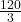## PLEASE HELP THIS IS URGENT Find the LARGER of the two, consecutive even numbers where twice the first plus the second is 122.

Question

PLEASE HELP THIS IS URGENT Find the LARGER of the two, consecutive even numbers where twice the first plus the second is 122.

in progress 0
6 months 2021-08-09T19:57:34+00:00 1 Answers 2 views 0

42

Step-by-step explanation:

2x + 2 + x = 122

3x = 122 – 2

3x = 120

x =x = 40.

If x is 40

Then x + 2 = 40 + 2

= 42

∴ 42 is the larger is the even numbers.